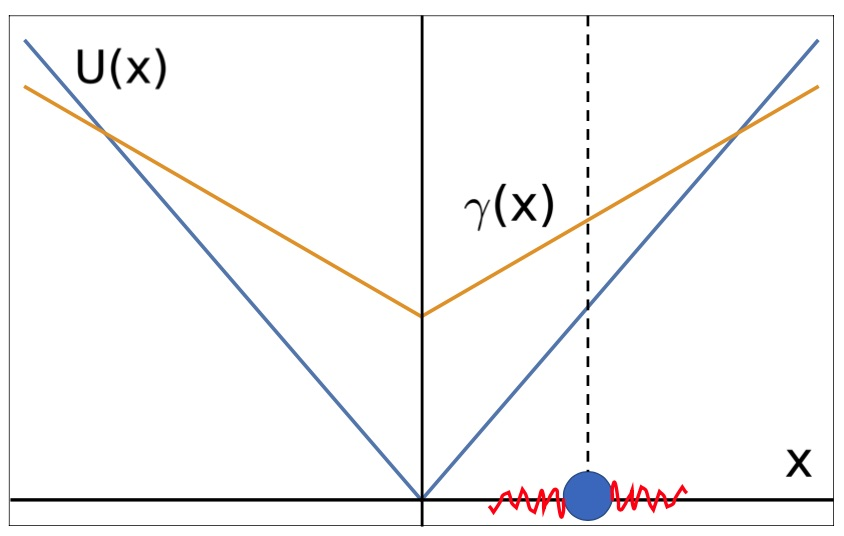## Brownian particles driven by spatially periodic noise published in EPJE

Brownian particles driven by spatially periodic noise
Davide Breoni, Ralf Blossey, Hartmut Löwen
The European Physical Journal E 45, 18 (2022)
arXiv: 2111.10220
DOI:10.1140/epje/s10189-022-00176-4

We discuss the dynamics of a Brownian particle under the influence of a spatially periodic noise strength in one dimension using analytical theory and computer simulations. In the absence of a deterministic force, the Langevin equation can be integrated formally exactly. We determine the short- and long-time behaviour of the mean displacement (MD) and mean-squared displacement (MSD). In particular we find a very slow dynamics for the mean displacement, scaling as t^(-1/2) with time t. Placed under an additional external periodic force near the critical tilt value we compute the stationary current obtained from the corresponding Fokker-Planck equation and identify an essential singularity if the minimum of the noise strength is zero. Finally, in order to further elucidate the effect of the random periodic driving on the diffusion process, we introduce a phase factor in the spatial noise with respect to the external periodic force and identify the value of the phase shift for which the random force exerts its strongest effect on the long-time drift velocity and diffusion coefficient.

## Active noise-driven particles under space-dependent friction in one dimension on arXivSketch of the confining potential U(x) = κ|x|, a linear friction gradient γ(x) = γ0+γ1|x| in arbitrary units. The particle, shown by a blue dot on the x-axis, is activated by noise (indicated in red), under the influence of the potential and the friction gradient. Image by D. Breoni.Active noise-driven particles under space-dependent friction in one dimension

Davide Breoni, Ralf Blossey, Hartmut Löwen
arxiv: 2102.09944

Abstract: We study a Langevin equation describing the stochastic motion of a particle in one dimension with coordinate x, which is simultaneously exposed to a space-dependent friction coefficient γ (x), a confining potential U(x) and non-equilibrium (i.e., active) noise. Specically, we consider frictions γ (x) = γ0 + γ1|x|p and potentials U(x) ∝ |x|p with exponents p = 1; 2 and n = 0; 1; 2. We provide analytical and numerical results for the particle dynamics for short times and the stationary
probability density functions (PDFs) for long times. The short-time behaviour displays diffusive and ballistic regimes while the stationary PDFs display unique characteristic features depending on the exponent values (p; n). The PDFs interpolate between Laplacian, Gaussian and bimodal distributions, whereby a change between these different behaviours can be achieved by a tuning of the friction strengths ratio
γ0 / γ1. Our model is relevant for molecular motors moving on a
one-dimensional track and can also be realized for confined self-propelled colloidal particles.

## Active Brownian and inertial particles in disordered environments: short-time expansion of the mean-square displacement on ArXiv

Active Brownian and inertial particles in disordered environments: short-time expansion of the mean-square displacement
Davide Breoni, Michael Schmiedeberg, Hartmut Löwen
arXiv: 2010.11076

We consider an active Brownian particle moving in a disordered two-dimensional energy or motility landscape. The averaged mean-square-displacement (MSD) of the particle is calculated analytically within a systematic short-time expansion. As a result, for overdamped particles, both an external random force field and disorder in the self-propulsion speed induce ballistic behaviour adding to the ballistic regime of an active particle with sharp self-propulsion speed. Spatial correlations in the force and motility landscape contribute only to the cubic and higher order powers in time for the MSD. Finally, for inertial particles two superballistic regimes are found where the scaling exponent of the MSD with time is α = 3 and α = 4. We confirm our theoretical predictions by computer simulations. Moreover they are verifiable in experiments on self-propelled colloids in random environments.